# Downsampling (decimating) a brain surface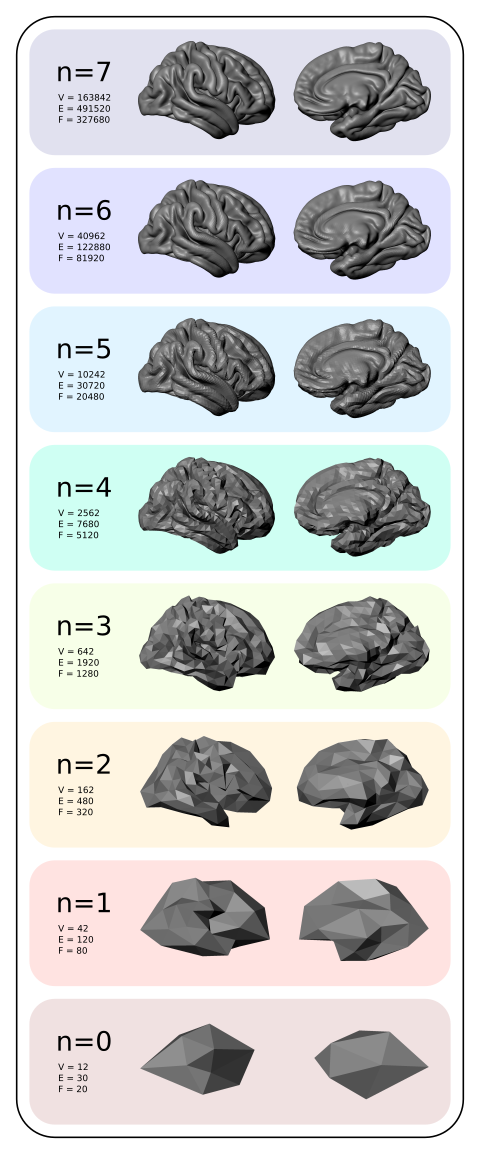Downsampled average cortical surfaces at different iterations (n), with the respective number of vertices (V), edges (E) and faces (F).

In the previous post, a method to display brain surfaces interactively in PDF documents was presented. While the method is already much more efficient than it was when it first appeared some years ago, the display of highly resolved meshes can be computationally intensive, and may make even the most enthusiastic readers give up even opening the file.

If the data being shown has low spatial frequency, an alternative way to display, which preserves generally the amount of information, is to decimate the mesh, downsampling it to a lower resolution. Although in theory this can be done in the native (subject-level) geometry through retessellation (i.e., interpolation of coordinates), the interest in downsampling usually is at the group level, in which case the subjects have all been interpolated to a common grid, which in general is a geodesic sphere produced by subdividing recursively an icosahedron (see this earlier post). If, at each iteration, the vertices and faces are added in a certain order (such as in FreeSurfer‘s fsaverage or in the one generated with the platonic command), downsampling is relatively straightforward, whatever is the type of data.

## Vertexwise data

For vertexwise data, downsampling can be based on the fact that vertices are added (appended) in a certain order as the icosahedron is constructed:

• Vertices 1-12 correspond to n = 0, i.e., no subdivision, or ico0.
• Vertices 13-42 correspond to the vertices that, once added to the ico0, make it ico1 (first iteration of subdivision, n = 1).
• Vertices 43-162 correspond to the vertices that, once added to ico1, make it ico2 (second iteration, n = 2).
• Vertices 163-642, likewise, make ico3.
• Vertices 643-2562 make ico4.
• Vertices 2563-10242 make ico5.
• Vertices 10243-40962 make ico6, etc.

Thus, if the data is vertexwise (also known as curvature, such as cortical thickness or curvature indices proper), the above information is sufficient to downsample the data: to reduce down to an ico3, for instance, all what one needs to do is to pick the vertices 1 through 642, ignoring 643 onwards.

## Facewise data

Data stored at each face (triangle) generally correspond to areal quantities, that require mass conservation. For both fsaverage and platonic icosahedrons, the faces are added in a particular order such that, at each iteration of the subdivision, a given face index is replaced in situ for four other faces: one can simply collapse (via sum or average) the data of every four faces into a new one.

## Surface geometry

If the objective is to decimate the surface geometry, i.e., the mesh itself, as opposed to quantities assigned to vertices or faces, one can use similar steps:

1. Select the vertices from the first up to the last vertex of the icosahedron in the level needed.
2. Iteratively downsample the face indices by selecting first those that are formed by three vertices that were appended for the current iteration, then for those that have two vertices appended in the current iteration, then connecting the remaining three vertices to form a new, larger face.

## Applications

Using downsampled data is useful not only to display meshes in PDF documents, but also, some analyses may not require a high resolution as the default mesh (ico7), particularly for processes that vary smoothly across the cortex, such as cortical thickness. Using a lower resolution mesh can be just as informative, while operating at a fraction of the computational cost.

## A script

A script that does the tasks above using Matlab/Octave is here: icodown.m. It is also available as part of the areal package described here, which also satisfies all its dependencies. Input and output formats are described here.

# Fast surface smoothing on a common grid

Smoothing scalar data on the surface of a high resolution sphere can be a slow process. If the filter is not truncated, the distances between all the vertices or barycentres of faces need to be calculated, in a very time consuming process. If the filter is truncated, the process can be faster, but with resolutions typically used in brain imaging, it can still be very slow, a problem that is amplified if data from many subjects are analysed.

However, if the data for each subject have already been interpolated to a common grid, such as an icosahedron recursively subdivided multiple times (details here), then the distances do not need to be calculated repeatedly for each of them. Doing so just once suffices. Furthermore, the implementation of the filter itself can be made in such a way that the smoothing process can be performed as a single matrix multiplication.

Consider the smoothing defined in Lombardi, (2002), which we used in Winkler et al., (2012):$\tilde{Q}_n = \dfrac{\sum_j Q_j K\left(g\left(\mathbf{x}_n,\mathbf{x}_j\right)\right)}{\sum_j K\left(g\left(\mathbf{x}_n,\mathbf{x}_j\right)\right)}$

where$\tilde{Q}_n$ is the smoothed quantity at the vertex or face$n$,$Q_j$ is the quantity assigned to the$j$-th vertex or face of a sphere containining$J$ vertices or faces,$g\left(\mathbf{x}_n,\mathbf{x}_j\right)$ is the geodesic distance between vertices or faces with coordinates$\mathbf{x}_n$ and$\mathbf{x}_j$, and$K(g)$ is the Gaussian filter, defined as a function of the geodesic distance between points.

The above formula requires that all distances between the current vertex or face$n$ and the other points$j$ are calculated, and that this is repeated for each$n$, in a time consuming process that needs to be repeated over again for every additional subject. If, however, the distances$g$ are known and organised into a distance matrix$\mathbf{G}$, the filter can take the form of a matrix of the same size,$\mathbf{K}$, with the values at each row scaled so as to add up to unity, and the same smoothing can proceed as a simple matrix multiplication:$\mathbf{\tilde{Q}} = \mathbf{K}\mathbf{Q}$

If the grid is the same for all subjects, which is the typical case when comparisons across subjects are performed,$\mathbf{K}$ can be calculated just once, saved, and reused for each subject.

It should be noted, however, that although running faster, the method requires much more memory. For a filter of full-width at half-maximum (FWHM)$f$, truncated at a distance$t \cdot f$ from the filter center, in a sphere of radius$r$, the number of non-zero elements in$\mathbf{K}$ is approximately:$\text{nnz} \approx \dfrac{J^2}{2} \left(1-\cos\left(t \cdot \dfrac{f}{r}\right)\right)$

whereas the total number of elements is$J^2$. Even using sparse matrices, this may require a large amount of memory space. For practical purposes, a filter with width$f$ = 20 mm can be truncated at twice the width ($t$ = 2), for application in a sphere with 100 mm made by 7 subdivisions of an icosahedron, still comfortably in a computer with 16GB of RAM. Wider filters may require more memory to run.

The script smoothdpx, part of the areal interpolation tools, available here, can be used to do both things, that is, smooth the data for any given subject, and also save the filter so that it can be reused with other subjects. To apply a previously saved filter, the rpncalc can be used. These commands require Octave or MATLAB, and if Octave is available, they can be executed directly from the command line.

## Figures

The figures above represent facewise data on the surface of a sphere of 100 mm radius, made by recursive subdivision of a regular icosahedron 4 times, constructed with the platonic command (details here), shown without smoothing, and smoothed with filters with FWHM = 7, 14, 21, 28 and 35 mm.

# Displaying vertexwise and facewise brain maps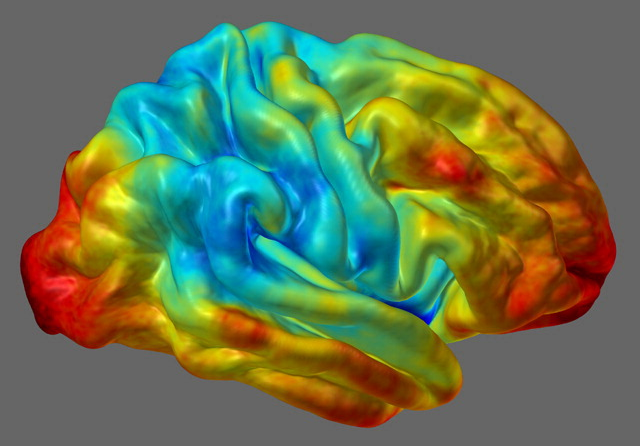In a previous post, a method to display FreeSurfer cortical regions in arbitrary colours was presented. Suppose that, instead, you would like to display the results from vertexwise or facewise analyses. For vertexwise, these can be shown using tksurfer or Freeview. The same does not apply, however, to facewise data, which, at the time of this writing, is not available in any neuroimaging software. In this article a tool to generate files with facewise or vertexwise data is provided, along with some simple examples.

## The dpx2map tool

The tool to generate the maps is dpx2map (right-click to download, then make it executable). Call it without arguments to get usage information. This tool uses Octave as the backend, and it assumes that it is installed in its usual location (/usr/bin/octave). It is also possible to run it from inside Octave or Matlab using a slight variant, dpx2map.m (in which case, type help dpx2map for usage).

In either case, the commands srfread, dpxread and mtlwrite must be available. These are part of the areal package discussed here. And yes, dpx2map is now included in the latest release of the package too.

To use dpx2map, you need to specify a surface object that will provide the geometry on which the data colours will be overlaid, and the data itself. The surface should be in FreeSurfer format (*.asc or *.srf), and the data should be in FreeSurfer “curvature” format (*.asc, *.dpv) for vertexwise, or in facewise format (*.dpf). A description of these formats is available here.

It is possible to specify the data range that will be used when computing the scaling to make the colours, as well which range will be actually shown. It is also possible to split the scale so that a central part of it is omitted or shown in a colour outside the colourscale. This is useful to show thresholded positive and negative maps.

The output is saved either in Stanford Polygon (*.ply) for vertexwise, or in Wavefront Object (*.obj + *.mtl) for facewise data, and can be imported directly in many computer graphics software. All input and output files must be/are in their respective ascii versions, not binary. The command also outputs a image with the colourbar, in Portable Network Graphics format (*.png).

## An example object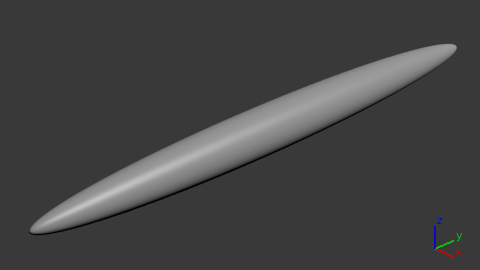With a simple geometric shape as this it is much simpler to demonstrate how to generate the maps, than using a complicated object as the brain. The strategy for colouring remains the same. For the next examples, an ellipsoid was created using the platonic command. The command line used was:

platonic ellipsoid.obj ico sph 7 '[.25 0 0 0; 0 3 0 0; 0 0 .25 0; 0 0 0 1]'


This ellipsoid has maximum y-coordinate equal to 3, and a maximum x- and z-coordinates equal to 0.25. This file was converted from Wavefront *.obj to FreeSurfer ascii, and scalar fields simply describing the coordinates (x,y,z), were created with:

obj2srf ellipsoid.obj > ellipsoid.srf
srf2area ellipsoid.srf ellipsoid.dpv dpv
gawk '{print $1,$2,$3,$4,$2}' ellipsoid.dpv > ellipsoid-x.dpv gawk '{print$1,$2,$3,$4,$3}' ellipsoid.dpv > ellipsoid-y.dpv
gawk '{print $1,$2,$3,$4,\$4}' ellipsoid.dpv > ellipsoid-z.dpv


It is the ellipsoid-y.dpv that is used for the next examples.

## Vertexwise examples

The examples below use the same surface (*.srf) and the same curvature, data-per-vertex file (*.dpv). The only differences are the way as the map is generated and presented, using different colour maps and different scaling. The jet colour map is the same available in Octave and Matlab. The coolhot5 is a custom colour map that will be made available, along with a few others, in another article to be posted soon.

### Example A

In this example, defaults are used. The input files are specified, along with a prefix (exA) to be used to name the output files.

dpx2map ellipsoid-y.dpv ellipsoid.srf exA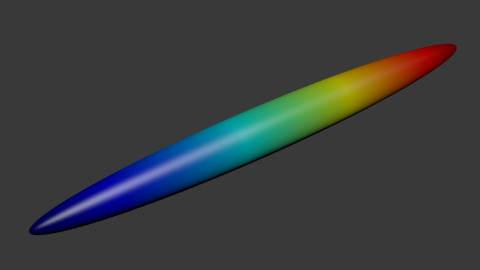### Example B

In this example, the data between values -1.5 and 1.5 is coloured, and the remaining receive the colours of the extreme points (dark blue and dark red).

dpx2map ellipsoid-y.dpv ellipsoid.srf exB jet '[-1.5 1.5]'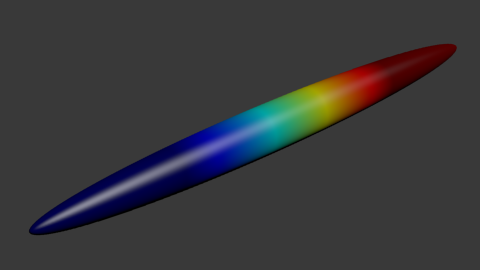### Example C

In this example, the data between -2 and 2 is used to define the colours, with the values below/above receiving the extreme colours. However, the range between -1 and 1 is not shown or used for the colour scaling. This is because the dual option is set as true as well as the coption.

dpx2map ellipsoid-y.dpv ellipsoid.srf exC coolhot5 '[-2 2]' '[-1 1]' true '[.75 .75 .75]' true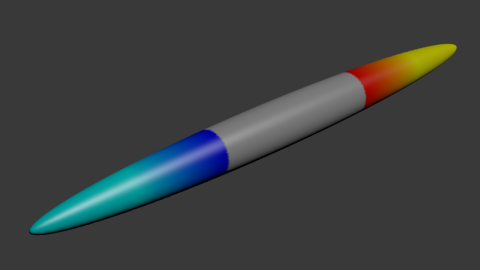### Example D

This example is similar as above, except that the values between -1 and 1, despite not being shown, are used for the scaling of the colours. This is due to the coption being set as true.

dpx2map ellipsoid-y.dpv ellipsoid.srf exD coolhot5 '[-2 2]' '[-1 1]' true '[.75 .75 .75]' false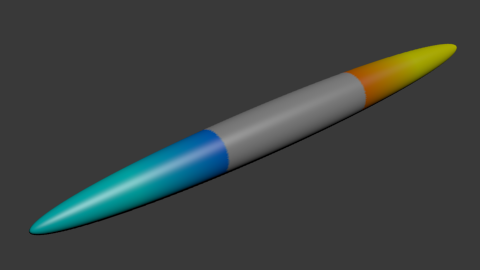### Example E

Here the data between -2 and 2 is used for scaling, but only the points between -1 and 1 are shown. This is because the option dual was set as false. The values below -1 or above 1 receive the same colours as these numbers, because the coption was configured as true. Note that because all points will receive some colour, it is not necessary to define the colourgap.

dpx2map ellipsoid-y.dpv ellipsoid.srf exE coolhot5 '[-2 2]' '[-1 1]' false '[]' true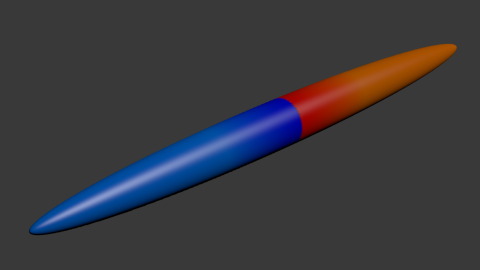### Example F

This is similar as the previous example, except that the values between -1 and 1 receive a colour off of the colour map. This is because both dual and coption were set as false.

dpx2map ellipsoid-y.dpv ellipsoid.srf exF coolhot5 '[-2 2]' '[-1 1]' false '[.75 .75 .75]' false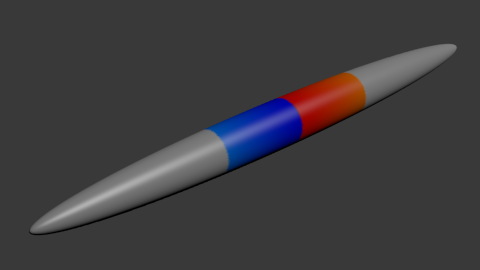## Facewise data

The process to display facewise data is virtually identical. The only two differences are that (1) instead of supplying a *.dpv file, a *.dpf file is given to the script as input, and (2) the output isn’t a *.ply file, but instead a pair of files *.obj + *.mtl. Note that very few software can handle thousands of colours per object in the case of facewise data. Blender is recommended over most commercial products specially for this reason (and of course, it is free, as in freedom).

The dpx2map is available here, and it is also included in the areal package, described here, where all its dependencies are satisfied. You must have Octave (free) or Matlab available to use this tool.

## How to cite

If you use dpx2map for your scientific research, please, remember to mention the brainder.org website in your paper.

## Update: Display in PDF documents

3D models as these, with vertexwise colours, can be shown in interactive PDF documents. Details here.

# Facewise brain cortical surface area: scripts now availableIn the paper Measuring and comparing brain cortical surface area and other areal quantities (Neuroimage, 2012;61(4):1428-43, doi:10.1016/j.neuroimage.2012.03.026, pmid:22446492), we described the steps to perform pycnophylactic interpolation, i.e., mass-conservative interpolation, of brain surface area. The method is also valid for any other quantity that is also areal by nature.

The scripts to perform the steps we propose in the paper are now freely available for Octave and/or matlab. They can also be executed directly from the shell using Octave as the interpreter. In this case, multiple instances can be easily submitted to run in a cluster. Click here to dowload and for step-by-step instructions.